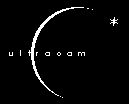0.0.0.0.0.0.0.0.1Document Title

Application Documentation

Document Number

Issue

1.1

Date

##### 0.0.0.0.1

 Document Prepared By: David Atkinson Signature and Date Document Approved By: Signature and Date Document Released By: David Lunney Signature and Date

CHANGE RECORD

 Issue Date Section affected Change Description

APPLICABLE DOCUMENTS

1. Introduction

This document describes the various CCD controller applications that can be run with ULTRACAM. All applications are downloaded to the SDSU timing board via the fibre link then executed with a ‘GO’ command. All applications reply to the 'GO' command with a 'RP' to indicate that the application has started, and all applications generate data. All image data packets are preceded by twelve 16-bit header words: 2 status words, 2 frame count words (24-bit count), and 8 words to hold time stamp information. Bit 0 of the first header word (status 1) is used to flag the last frame (or end of application), and bit 1 of same word is used to flag if the last frame was generated by a stop command 'ST'. All data packets include the two status words so that the end of the application can be flagged. For example Application 1 (power on) sends a packet of data that includes the status words only. Here, the 'last frame' flag is set to indicate that the application has finished.

Some of the applications have parameters, which can be passed to the timing board, e.g. the number of exposures or binning factors. All parameters are stored in the on-chip memory (location X:\$005A to X:\$0070) of the timing board’s DSP56002. Note that this document will represent a hexadecimal number by preceding it with the symbol ‘\$’.

All of the image data generating applications read out Ultracam's three chips in parallel, and parameters apply to all three CCDs. Furthermore, each chip has two readout channels that are always read out and sampled in parallel.

2. Application 1: POWER ON

This application causes the SDSU controller to switch power through to the video and clock boards in a controlled manner. After switching on the SDSU controller, the power on application must be downloaded and run once before any CCD readout application can be downloaded and executed. After a successful execution of the power on application all the LEDs on the power control board (at the back of the SDSU controller) should be on. This application has no parameters.

3. Application 2: POWER OFF

The execution of this application causes the SDSU to disable the voltages to the CCD and should be used at the end of an observation run. This application has no parameters.

4.1. Application 3: Description

This application is used to read out the entire CCD (1024 pixels x 1024 pixels).

4.2. Application 3: Parameters

There are six parameters for application 3, as described in Table 4.1 below.

###### 0.0.0.0.1.3Default

NO_EXPOSURES

The number of exposures taken each time the application is executed

1

EXPOSE_TIME

The frame exposure time in milliseconds

1000 (1s)

GAIN_SPEED

The gain and readout speed setting see Table 4.2 for various options

\$CDD (gives approx. 1.2e/DN at 10us per pixel)

X_BIN_FAC

X binning factor. Bins the number of columns.

1

Y_BIN_FAC

Y binning factor. Bins the number of rows.

1

V_FT_CLK

Frame Transfer V Clock timing parameter (described in Appendix A)

\$990000

Table 4.1: Full-Frame Application Parameters

Each parameter has limits on it and consequently invalid entries input by the user will be rejected and an error returned. The limits are described in Table 4.2.

###### 0.0.0.0.1.5Limits

NO_EXPOSURES

NO_EXPOSURES -1,

NO_EXPOSURES 0,

NO_EXPOSURES 65535

When this parameter is set to –1 the camera is put into “continual readout mode”, i.e. infinite number of exposures. This can only be terminated with a stop command ‘ST’ to the timing board.

EXPOSE_TIME

EXPOSE TIME 0

The exposure time is expressed in milliseconds

GAIN_SPEED

GAIN_SPEED = \$CEE, or GAIN_SPEED = \$CDD, or

GAIN_SPEED = \$CBB, or

GAIN_SPEED = \$C77, or

GAIN_SPEED = \$FEE, or

GAIN_SPEED = \$FDD, or

GAIN_SPEED = \$FBB, or

GAIN_SPEED = \$F77

As shown this parameter can only have one of 8 values. The gain refers to a variable gain stage setting on the SDSU video board.

GAIN SPEED

x 9.5 SLOW (10us/pix)

x 4.75 SLOW

x 2.0 SLOW

x 1.0 SLOW

x 9.5 FAST (2us/pix)

x 4.75 FAST

x 2.0 FAST

x 1.0 FAST

It is recommended that \$CDD or \$FDD be used. Both yield a system gain of approx. 1.2e/DN.

X_BIN_FAC

512 % X_BIN_FAC = 0

The number of columns (per channel) must exactly dividable by the X binning factor.

Y_BIN_FAC

1024 % Y_BIN_FAC = 0

The number of rows must exactly dividable by the Y binning factor.

V_FT_CLK

V_FT_CLK > \$260000

V_FT_CLK < \$FF0000

V_FT_CLK \$800000

16 least significant bits (0-15) of V_FT_CLK must equal 0

The most significant byte of this parameter determines the period of the V clocks used to perform a frame transfer. The two least significant bytes must both be \$00. See Appendix A full description

Table 4.2: Full-Frame Application Parameter Limits

This full-frame readout application clears the CCD before every exposure. Immediately after the CCD is cleared the DSP generates a timestamp interrupt to mark the beginning of the next exposure. The CCD is then exposed for the time provided by parameter EXPOSE_TIME. A frame transfer is then performed to shift the image area into the storage area. The cycle is then repeated for the number of times specified in parameter, 'NO_EXPOSURES'. This cycle is illustrated in Figure 4.1 below. Note that because the CCD is cleared before each exposure, the exposure time is exactly that specified by the parameter EXPOSE_TIME.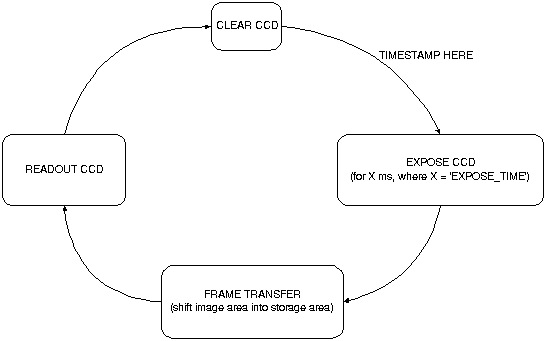As shown, this application clears the CCD before each exposure. Reading out a full frame takes several seconds, therefore, without clearing each time the minimum exposure time would be in the order of seconds. Therefore, to permit quicker exposure times the Clear CCD is included. However, this does mean there is a significant 'dead-time' in each cycle, where the CCD is integrating on unused signal. A second full-frame readout application without a clear CCD between successive frames is also available as is described in Section 10.

5. Application 4: FULL-FRAME READOUT with OVERSCAN

5.1. Application 4: Description

A full-frame contains 1024x1024 image pixels. However, in addition to this the CCD47-20 has 24 under-scan columns (8 blank element columns and 16 dark reference columns), 24 over-scan column (16 dark reference columns and 8 blank element columns) and 3 over-scan rows (dark reference rows). This yields a frame size of 1072 x 1027. Application 4 can be executed to obtain a full frame image with under-scan and over-scan rows and columns. In order to aid binning options an additional 8 blank over-scan columns and 5 over-scan rows will be clocked to yield a total frame size of 1080 x 1032. A typical image (with no binning) is pictorially illustrated in Figure 5.1. As indicated, the additional 8 columns will appear in the centre of the chip since it is being readout from both outputs.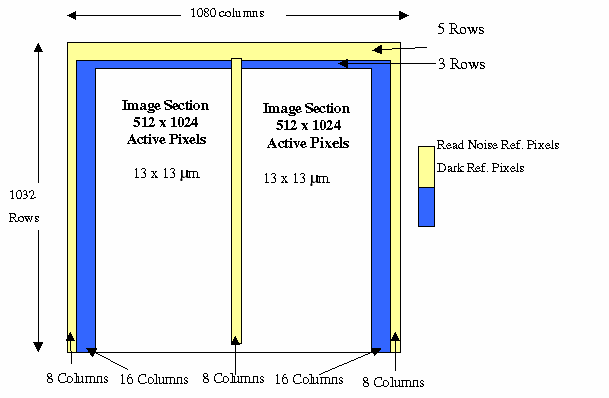5.2. Application 4: Parameters

The parameters for this application are the same as that of Application 3, and are listed in Tabled 4.1. The limits on these parameters are also the same as Application 3 (see Table 4.2), with the exception of X_BIN_FACTOR and Y_BIN_FACTOR, detailed in Table 5.1.

 X_BIN_FAC 540 % X_BIN_FAC = 0 The number of columns (per channel) must exactly dividable by the X binning factor. Y_BIN_FAC 1032 % Y_BIN_FAC = 0 The number of rows must exactly dividable by the Y binning factor.

Table 5.1: Application 4 parameter limits

As Application 3, see Figure 4.1.

6. Application 5: WINDOW READOUT – 1PAIR

6.1. Application 5: Description

This application allows 2 windows to be defined and readout per CCD frame. Window locations are specified from the first pixel in the image area. That is location 1,1 is the bottom left of the image area and location 1024,1024 is the top right of the image area. Again, under-scan and over-scan columns and rows are disregarded. The two windows should be thought of a window pair, with one window in each half of the CCD image area. That is one window (window 1L) in the left half of the chip (X = 1 to 512) and the other window (window 1R) in the right half of the chip (x = 513 to 1024). The windows will have the same starting position in Y and the same X and Y size dimensions.

6.2. Application 5: Parameters

In addition to the parameters described for application 3 (in Table 4.1) this application has various parameters that describe the window sizes and locations. The parameters are detailed below in Table 6.1.

###### 0.0.0.0.1.9Default

NO_EXPOSURES

The number of exposures taken each time the application is executed

1

EXPOSE_TIME

The frame exposure time in milliseconds

1000 (1s)

GAIN_SPEED

The gain and readout speed setting see Table 3.2 for various options

\$CDD

X_BIN_FAC

X binning factor. Bins the number of columns.

1

Y_BIN_FAC

Y binning factor. Bins the number of rows.

1

V_FT_CLK

Frame Transfer V Clock timing parameter (described in Appendix A)

\$990000

X1L_START

The x-axis starting position of window 1 Left.

100

X1R_START

The x-axis starting position of window 1 Right.

600

Y1_START

The y-axis starting position of window pair 1

200

X1_SIZE

The size in x-axis of window pair 1

60

Y1_SIZE

The size in y-axis of window pair 1

60

Table 6.1: Window Application (1 Pair) Parameters

As before, each parameter has limits on it and the camera object will generate an error when the user inputs an invalid value. The limits for this application’s parameters are described in Table 6.2.

###### 0.0.0.0.1.11Limits

NO_EXPOSURES

As Table 3.2

EXPOSE_TIME

As Table 3.2

GAIN_SPEED

As Table 3.2

X_BIN_FAC

X1_SIZE % X_BIN_FAC = 0

The X bin factor is the same for both windows.

Y_BIN_FAC

Y1_SIZE % Y_BIN_FAC = 0

The Y bin factor is the same for both windows.

V_FT_CLK

As Table 3.2

X1L_START

X1L_START 1, AND

X1L_START 512

X1R_START

X1R_START 513, AND

X1R_START 1024

Y1_START

Y1_START 1

Y1_START 1024

Influences both Left and Right windows

X1_SIZE

X1_SIZE 1, AND

X1_SIZE 513 – X1L_START, AND

X1_SIZE 1025 – X1R_START

Influences both Left and Right windows

Y1_SIZE

Y1_SIZE 1 AND

Y1_SIZE 1025 – Y1_START

Influences both Left and Right windows

Table 6.2: Window Application Parameter Limits

Unlike the previously described full frame applications, the window mode applications do not clear the CCD between successive readouts. In fact, the CCD is only cleared once, before the first exposure. Following this, each frame is exposed for the time specified by parameter EXPOSE_TIME, then shifted from the image area into the storage area to complete the exposure stage. It is here that the timestamp interrupt is performed marking the end of the current exposure and the beginning of the next exposure. The current frame is then readout of the storage area, during which time the next frame's exposure has begun. Once the readout is complete the cycle is repeated, as illustrated in Figure 6.1. Therefore, although the first frame has an exposure time equal to that specified by the parameter EXPOSE_TIME, all other frames have an exposure time equal to EXPOSE_TIME plus the time it takes to readout the previous frame T(Readout), as stated in Equation 6.1. Consequently, the first frame should be ignored in any observing run sequence.

Exposure Time = EXPOSE_TIME + T(Readout) (6.1)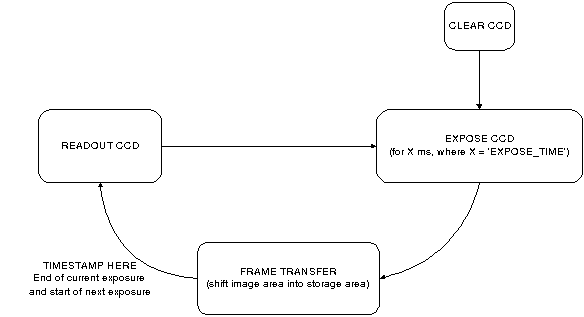Figure 6.1: Window Mode Readout Sequence

7. Application 6: WINDOW READOUT – 2PAIR

7.1. Application 6: Description

This Application is similar to Application 6, except four windows are specified. Again windows should be though of in pairs, therefore, 2 window pairs are defined. A window pair must have one window in the left half of the chip (X = 1 to 512) and the other window in the right half of the chip (x = 513 – 1024), the same starting position in the Y-axis, and the same X and Y dimensions. Windows cannot overlap in Y.

7.2. Application 6: Parameters

The available parameters for this application are described in Table 7.1.

###### 0.0.0.0.1.15Default

NO_EXPOSURES

The number of exposures taken each time the application is executed

1

EXPOSE_TIME

The frame exposure time in milliseconds

1000 (1s)

GAIN_SPEED

The gain and readout speed setting see Table 3.2 for various options

\$CDD

X_BIN_FAC

X binning factor. Bins the number of columns.

1

Y_BIN_FAC

Y binning factor. Bins the number of rows.

1

V_FT_CLK

Frame Transfer V Clock timing parameter (described in Appendix A)

\$990000

X1L_START

The x-axis starting position of window 1 Left.

100

X1R_START

The x-axis starting position of window 1 Right.

600

Y1_START

The y-axis starting position of window pair 1

200

X1_SIZE

The size in x-axis of window pair 1

120

Y1_SIZE

The size in y-axis of window pair 1

120

X2L_START

The x-axis starting position of window 2 Left.

200

X2R_START

The x-axis starting position of window 2 Right.

700

Y2_START

The y-axis starting position of window pair 2

600

X2_SIZE

The size in x-axis of window pair 2

120

Y2_SIZE

The size in y-axis of window pair 2

120

Table 7.1: Window Application (2 Pairs) Parameters

The limits for this application’s parameters are described in Table 7.2.

###### 0.0.0.0.1.17Limits

NO_EXPOSURES

As Table 3.2

EXPOSE_TIME

As Table 3.2

GAIN_SPEED

As Table 3.2

X_BIN_FAC

X1_SIZE % X_BIN_FAC = 0 AND

X2_SIZE % X_BIN_FAC = 0

The X bin factor is the same for all the windows.

Y_BIN_FAC

Y1_SIZE % Y_BIN_FAC = 0 AND

Y2_SIZE % Y_BIN_FAC = 0

The Y bin factor is the same for all windows.

###### 0.0.0.0.1.19V_FT_CLK

As Table 3.2

X1L_START

X1L_START 1, AND

X1L_START 512

X1R_START

X1R_START 513, AND

X1R_START 1024

Y1_START

Y1_START 1

Y1_START 1024

Influences both Left and Right windows

X1_SIZE

X1_SIZE 1, AND

X1_SIZE 513 – X1L_START, AND

X1_SIZE 1025 – X1R_START

Influences both Left and Right windows

Y1_SIZE

Y1_SIZE 1 AND

Y1_SIZE 1025 – Y1_START

Influences both Left and Right windows

X2L_START

X2L_START 1, AND

X2L_START 512

X2R_START

X2R_START 513, AND

X2R_START 1024

Y2_START

Y2_START Y1_START+Y1_SIZE, AND

Y2_START 1024

Influences both Left and Right windows

X2_SIZE

X2_SIZE 1, AND

X2_SIZE 513 – X2L_START

X2_SIZE 1025 – X2R_START

Influences both Left and Right windows

Y2_SIZE

Y2_SIZE 1 AND

Y2_SIZE 1025 – Y2_START

Influences both Left and Right windows

Table 7.2: Window Application (2 Pairs) Parameter Limits

This follows the same model as Application 5 (see Section 6.1) except a frame now consists of four windows.

8. Application 7: WINDOW READOUT – 3PAIR

8.1. Application 7: Description

This Application can be used to read out 6 windows per frame. As before, windows should be though of in pairs, therefore, three window pairs are defined here. A window pair must have one window in the left half of the chip (X = 1 to 512) and the other window in the right half of the chip (x = 513 – 1024), the same starting position in the Y-axis, and the same X and Y dimensions. Windows cannot overlap in Y.

8.2. Application 7: Parameters

The available parameters for this application are described in Table 8.1.

###### 0.0.0.0.1.22Default

NO_EXPOSURES

The number of exposures taken each time the application is executed

1

EXPOSE_TIME

The frame exposure time in milliseconds

1000 (1s)

GAIN_SPEED

The gain and readout speed setting see Table 3.2 for various options

\$CDD

X_BIN_FAC

X binning factor. Bins the number of columns.

1

Y_BIN_FAC

Y binning factor. Bins the number of rows.

1

V_FT_CLK

Frame Transfer V Clock timing parameter (described in Appendix A)

\$990000

X1L_START

The x-axis starting position of window 1 Left.

290

X1R_START

The x-axis starting position of window 1 Right.

750

Y1_START

The y-axis starting position of window pair 1

150

X1_SIZE

The size in x-axis of window pair 1

80

Y1_SIZE

The size in y-axis of window pair 1

80

X2L_START

The x-axis starting position of window 2 Left.

270

X2R_START

The x-axis starting position of window 2 Right.

730

Y2_START

The y-axis starting position of window pair 2

540

X2_SIZE

The size in x-axis of window pair 2

80

Y2_SIZE

The size in y-axis of window pair 2

80

X3L_START

The x-axis starting position of window 3 Left.

4

X3R_START

The x-axis starting position of window 3 Right.

750

Y3_START

The y-axis starting position of window pair 3

675

X3_SIZE

The size in x-axis of window pair 3

80

Y3_SIZE

The size in y-axis of window pair 3

80

Table 8.1: Window Application (3 Pairs) Parameters

The limits for this application’s parameters are described in Table 8.2.

###### 0.0.0.0.1.24Limits

NO_EXPOSURES

As Table 3.2

EXPOSE_TIME

As Table 3.2

GAIN_SPEED

As Table 3.2

X_BIN_FAC

X1_SIZE % X_BIN_FAC = 0, AND

X2_SIZE % X_BIN_FAC = 0, AND

X3_SIZE % X_BIN_FAC = 0, AND

The X bin factor is the same for all the windows.

Y_BIN_FAC

Y1_SIZE % Y_BIN_FAC = 0, and

Y2_SIZE % Y_BIN_FAC = 0, and

Y3_SIZE % Y_BIN_FAC = 0, and

The Y bin factor is the same for all the windows

###### 0.0.0.0.1.26V_FT_CLK

As Table 3.2

X1L_START

X1L_START 1, AND

X1L_START 512

X1R_START

X1R_START 513, AND

X1R_START 1024

Y1_START

Y1_START 1

Y1_START 1024

Influences both Left and Right windows

X1_SIZE

X1_SIZE 1, AND

X1_SIZE 513 – X1L_START, AND

X1_SIZE 1025 – X1R_START

Influences both Left and Right windows

Y1_SIZE

Y1_SIZE 1 AND

Y1_SIZE 1025 – Y1_START

Influences both Left and Right windows

X2L_START

X2L_START 1, AND

X2L_START 512

X2R_START

X2R_START 513, AND

X2R_START 1024

Y2_START

Y2_START Y1_START+Y1_SIZE, AND

Y2_START 1024

Influences both Left and Right windows

X2_SIZE

X2_SIZE 1, AND

X2_SIZE 513 – X2L_START

X2_SIZE 1025 – X2R_START

Influences both Left and Right windows

Y2_SIZE

Y2_SIZE 1 AND

Y2_SIZE 1025 – Y2_START

Influences both Left and Right windows

X3L_START

X3L_START 1, AND

X3L_START 512

X3R_START

X3R_START 513, AND

X3R_START 1024

Y3_START

Y3_START Y2_START+Y2_SIZE, AND

Y3_START 1024

Influences both Left and Right windows

X3_SIZE

X3_SIZE 1, AND

X3_SIZE 513 – X3L_START

X3_SIZE 1025 – X3R_START

Influences both Left and Right windows

Y3_SIZE

Y3_SIZE 1 AND

Y3_SIZE 1025 – Y3_START

Influences both Left and Right windows

Table 8.2: Window Application (3 Pairs) Parameter Limits

This follows the same model as Application 5 (see Section 6.1) except a frame now consists of four windows.

9. Application 8: Drift-scan Mode

9.1. Application 8: Description

Driftscan mode is used for high-speed readout operation. It continuously reads out two similar windows with the same Y_START position, one from each half of the chip. The CCD storage area is used to pipeline a stack of exposed windows before they are readout, thereby minimising the readout 'dead-time'. Typically, a window pair is exposed then quickly shifted from the CCD's image area into the top of its storage area for the next exposure to begin. Consequently, for optimum temporal performance the window pair should be located as close to the image/storage area boundary as possible (i.e. have a small Y1_START parameter). During a given exposure the window pair at the end of the storage area pipeline is readout. On reading out a window pair all the windows in the storage area are shifted down the chip by the number of rows in a window (Y1_SIZE). Consequently, when the next window pair is shifted into storage area it will have Y1_SIZE rows between it and the previous window pair. The number of window pairs (NO_WINS) permitted in the pipeline is dependant on the size of the window and the size of the storage area. . Unfortunately, the CCD47-20 has 1033 rows in its storage area, which means an exact number of windows (and separations) cannot be stacked in the pipeline (since 1033 is a prime number). To overcome this extra shifts are required once the pipeline is full to shunt the stack of window pairs to the bottom of the storage area. For a given window size (Y1_SIZE), the number of window pairs that can be stacked in the pipeline is calculated from Equation 9.1., (9.1)

(Floor returns the nearest integer value below the calculated real number.)

Therefore, the extra number of required shifts (once the pipeline is full) is given by: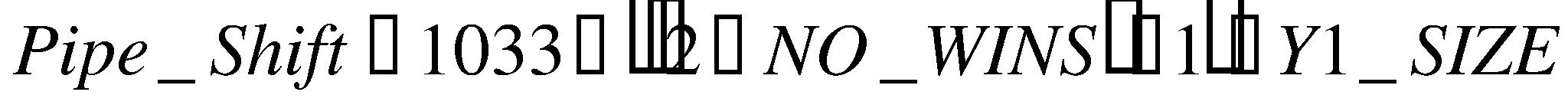(9.2)

This additional number of shifts is performed before every Nth window readout, where N = NO_WINS.

9.3. Application 8: Parameters

The parameters used by this application are detailed in Table 9.1.

###### 0.0.0.0.1.29Default

NO_EXPOSURES

The number of exposures taken each time the application is executed

1

EXPOSE_TIME

The frame exposure time in milliseconds

1000 (1s)

GAIN_SPEED

The gain and readout speed setting see Table 3.2 for various options

\$CDD

X_BIN_FAC

X binning factor. Bins the number of columns.

1

Y_BIN_FAC

Y binning factor. Bins the number of rows.

1

V_FT_CLK

Frame Transfer V Clock timing parameter (described in Appendix A)

\$990000

X1L_START

The x-axis starting position of window 1 Left.

100

X1R_START

The x-axis starting position of window 1 Right.

600

Y1_START

The y-axis starting position of window pair 1

1

X1_SIZE

The size in x-axis of window pair 1

49

Y1_SIZE

The size in y-axis of window pair 1

49

Table 9.1: Window Application (1 Pair) Parameters

As before, each parameter has limits on it and the camera object will generate an error when the user inputs an invalid value. The limits for this application’s parameters are described in Table 9.2.

###### 0.0.0.0.1.31Limits

NO_EXPOSURES

As Table 3.2

EXPOSE_TIME

As Table 3.2

GAIN_SPEED

As Table 3.2

X_BIN_FAC

X1_SIZE % X_BIN_FAC = 0

The X bin factor is the same for both windows.

Y_BIN_FAC

Y1_SIZE % Y_BIN_FAC = 0

The Y bin factor is the same for both windows.

V_FT_CLK

As Table 3.2

X1L_START

X1L_START 1, AND

X1L_START 512

Left Hand of Chip

X1R_START

X1R_START 513, AND

X1R_START 1024

Right Hand of Chip

Y1_START 1

Y1_START 1024

Influences both Left and Right windows

X1_SIZE

X1_SIZE 1, AND

X1_SIZE 513 – X1L_START, AND

X1_SIZE 1025 – X1R_START

Y1_SIZE

Y1_SIZE 1 AND

Y1_SIZE 1025 – Y1_START

See Table 9.3 for optimum values. Recommended values are 24, 49, 54, 79 114, and 147

Table 9.2: Window Application Parameter Limits

9.3. Application 8: Driftscan mode example detailing readout sequence

Consider a window pair with 160 rows each (Y1_SIZE=160). This is a rather large window for Driftscan mode but is used here for example purposes. From Equation 9.1 the number of permitted window pairs in the pipeline is:

NO_WINS = floor (3.73) = 3

Consequently, from Equation 9.2 the Pipe-shift required before every Nth readout is:

Pipe_shift = 233

Therefore, an extra 233 Vertical clocks are required on the storage area before every 3rd window is readout. This is pictorially illustrated in Figure 9.1. Step one shows an exposure being taken on window pair 1. After a specified exposure time (specified by parameter EXPOSE_TIME) the window pair is shifted into the top of the storage area. This is only shown as one step here, however it is actually done in two stages: firstly the window pair is shifted to the bottom of the image area using the Image V clocks only, then the window pair is shifted into the storage area using the Storage and Image V clocks. Consequently, everything in the storage area is shifted downwards by the number of rows in the window (160 for this case). As soon as window pair 1 has been shifted into the Storage area the exposure begins on window pair 2. It is at this stage that window pair 1 is time-stamped. While the exposure on window pair 2 is taking place, the window pair at the end of the pipeline is readout. At this stage the end of the pipeline does not contain any real image data, and step 3 of Figure 1 illustrates this with 'garbage' window pair A being readout. This cycle continues until the pipeline is full, i.e. step 8 of Figure 1, at this point the pipeline needs to be shifted (in this case 233 times) to get window pair 1 to the bottom of the Storage area ready for readout. Window pair 1 is then readout while exposing window pair 4, and the cycle continues.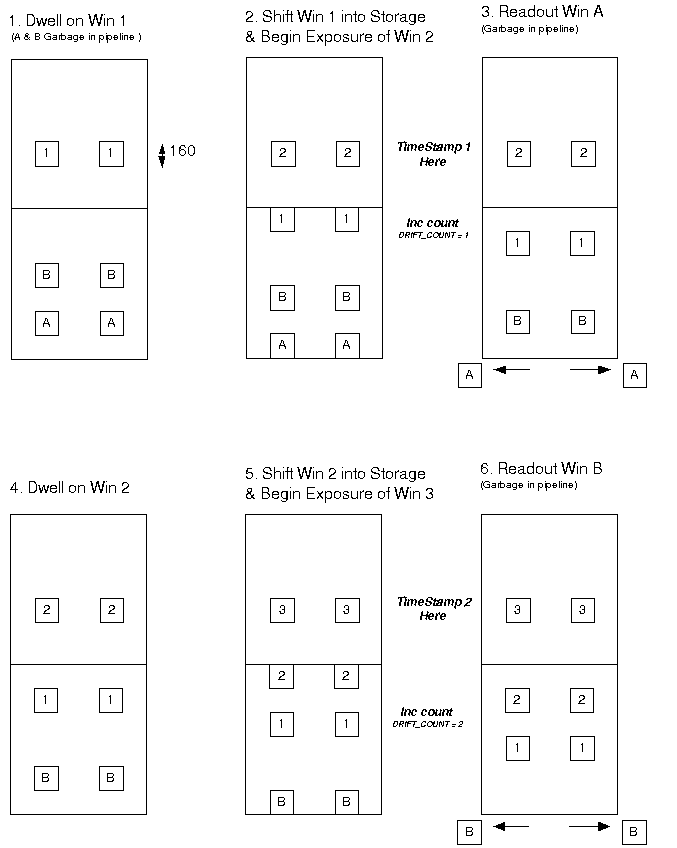Figure 1: Driftscan Readout with 3 windows in Pipeline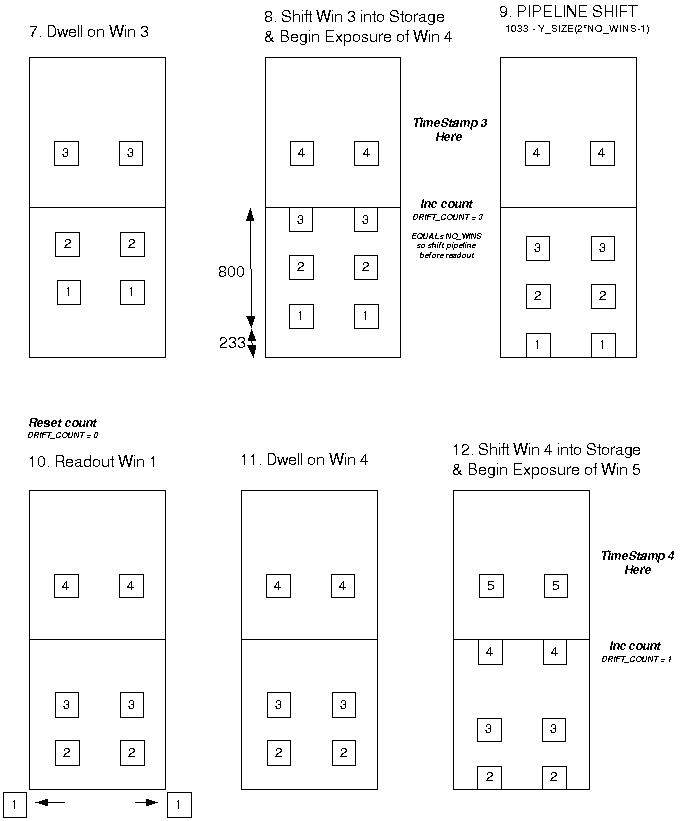Figure 1 (cont.): Driftscan Readout with 3 windows in Pipeline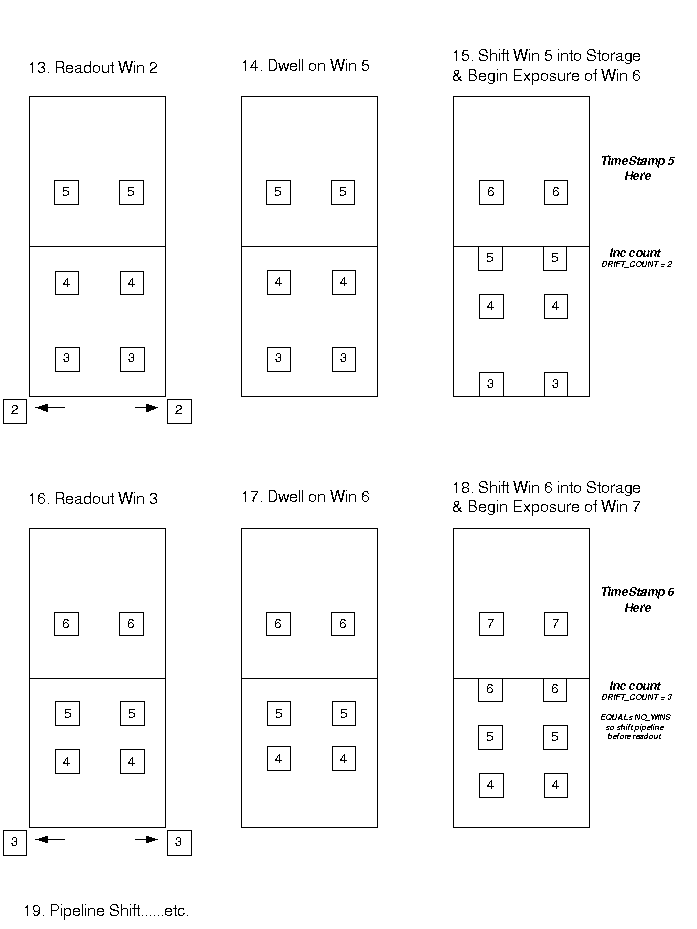Figure 9.1 (cont.): Driftscan Readout with 3 windows in Pipeline

It can be seen from this example that at the beginning of any run the first few window pairs will contain garbage. The number of garbage windows, NWIN(Garbage), is equal to the number of windows that can be stacked in the pipeline, N_WINS, minus one, as stated in Equation 9.3. Solving Equation 9.3 for the above example yields NWINS(Garbage) = 2, which were represented by window pairs A and B. Note that NWINS(garbage) also provides the offset between the timestamp and the associated window. For example window pair 1 is readout just after timestamp 3 is generated.

NWINS(Garbage) = N_WINS - 1 (9.3)

= Timestamp Offset

9.3. Application 8: Optimisation of windows

In the previous example an extra 233 V clocks were required before every third readout. This represents a significant overhead, for example, with V clocks of 24us this extra shift would take 5.6ms, thereby crippling the fastest readout speeds. Moreover, this additional delay will add to the exposure time of the window in the image area during the shift time, and so every third window would have a 5.6ms extended exposure time. Ideally, this number should be zero, however this could only be the case if an exact number of windows (and separations) could be stacked in the storage area, which is impossible for this CCD size. A plot of window size versus required shift is provided in Figure 9.2 revealing that careful choice of window size will minimise the amount of extra shifts required. The minima of the plot are presented in Table 9.1.Figure 9.2: Pipeline shifts vs. window Y1_SIZE

 Y1_SIZE N_WINS (N) Pipe_Shift (before Nth readout 8 65 1 10 52 3 13 40 6 18 29 7 21 25 4 24 22 1 31 17 10 38 14 7 41 13 8 49 11 4 54 10 7 60 9 13 68 8 13 79 7 6 93 6 10 114 5 7 147 4 4 206 3 3 344 2 1

Table 9.1: Minimum Pipe Shifts

Consequently, for minimum extra shift times these minima values should be selected for the Y1_SIZE parameter. It is recommended that that the following six window values be used: 24, 49, 54, 79 114, and 147, providing a reasonable range of window size options, and a maximum pipe shift time of 168us (with the current V clock rate of 24us/pix). Consequently, the maximum increase in exposure time every Nth window would be approximately 1.6% at a 100Hz readout rate. However, for faster readout speeds even this small delay could become significant yielding an erroneous signal in the power spectrum of the measured light curve. In order to overcome this, an additional delay equal to the shift time will be added to all other exposures, resulting in all frames having an equal exposure time. The readout sequence diagram detailed in Figure 9.3 illustrates this. Consequently, all frames have an exposure time equal to that specified (in ms) by the parameter EXPOSE_TIME, plus the time it takes to readout a window pair T(Readout), plus the shunt pipeline delay T(Pipe_Shunt), as stated in Equation 9.4.Figure 9.3: Driftscan mode Readout Sequence

Exposure Time = EXPOSE_TIME + T(Readout) + T(Pipe_Shunt) (9.4)

Where, T(Pipe_Shunt) = Pipe_Shift * T(V_Clock).

= Pipe_Shift * 24us.

Pipe_Shift is calculated from Equation 9.2, and the minima are listed in Table 9.2.

Note on window location in Y-axis

Recall that for optimum readout speeds the window pair location should be chosen to be as close the bottom of the image area as possible so that it can be quickly shifted into the storage area.

10. Application 9: Full Frame with Minimum Dead-time

10.1. Application 9: Description

As with Application 3, this mode reads out the entire CCD without overscan (1024 pixels x 1024 pixels). However, in this mode the CCD is only cleared once at the beginning of the run. Consequently, it follows a similar timing model to Applications 5, 6, and 7 (window modes), as illustrated in Figure 6.1. Essentially, this results in a full frame readout mode with minimum 'dead-time' since the chip is integrating on the next frame while reading out the current frame. However, it does limit the minimum total exposure time to that of a full frame readout.

10.2. Application 9: Parameters

The parameters are the same as those for Application 3 as described in Section 4. re are five parameters for application 3, as described in Table 4.1 below.

As with the window readout modes the total exposure time includes the time it takes to readout the previous frame. Consequently, the readout time is given by Equation 10.1. Note that the frame transfer time is ignored since is negligible compared with T(Readout) for full frame.

Exposure Time = EXPOSE_TIME + T(Readout) (10.1)

Appendix A: Frame Transfer V Clock Parameter

The 24-bit parameter 'V_FT_CLK' is used to set a delay, which determines the period of the Vertical Clocks used to perform a frame transfer (FT). The period of the FT clocks is exactly 6 times the delay specified by V_FT_CLK.

The value of this delay is calculated from the most significant byte of the parameter and is equal to:

Delay = 80ns + (fine/course adjustment) x multiplier

Where the most significant bit (bit 23) determines the fine/course adjustment and bits 16-22 give the multiplier.

If bit 23 = 0, then the fine/course adjust is 20ns.

If bit 23 = 1, then the fine/course adjust is 160ns.

Note that the two least two significant bytes (bits 0 to 15) of V_FT_CLK must both be \$00.

Some example parameter values and equivalent delays and clock periods are provided in Table A.1.

 V_FT_CLK Delay FT V Clock Period \$260000 80ns+20ns*38 = 840ns s \$360000 80ns+20ns*54 = 1160ns s \$4F0000 80ns+20ns*79 = 1680ns s \$790000 80ns+20ns*121 = 2500ns s \$990000 80ns+160ns*25 = 4080ns 24.5s \$800000 NOT PERMITTED NOT PERMITTED

Table A.1: Example values of V_FT_CLKApplication_Documentation_R1.0.doc